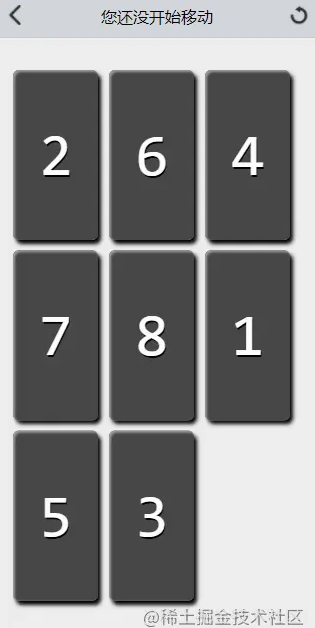﻿ 基于Vue3实现数字华容道游戏的示例代码_vue.js_脚本之家
vue.js# 基于Vue3实现数字华容道游戏的示例代码

## 环境

vue3 version：3.2.4

vite version：2.5.0

vue-router version：4.0.14

windicss version：3.5.1## 思路

• 搭建环境，下载依赖
• 运行项目
• 利用windicss主体兼容pc和移动端

App.vue

```<div class="relative w-full h-full lg:(w-750px h-800px)">
<route-view
</div>
```

## 实现

### GameCnt

• 先画出3*3的格子，这里有多种方法，笔者这里采取最简单的动态grid布局实现，后来因为css动画选取的是transform则不用gird布局了
• 宽高获取,这里要获取，原因是使用了transform位移动画，则需要平移距离和宽高了
• 设置lazyShow，让第一次渲染不会有transform动画
• 隐藏最后一个，利用数组对象value值+css实现
• 添加其他css（windicss不好实现的css）
```// 定义行个数
const rowLen = 3
// 定义cnt宽高和item的宽高
const cntWidth = ref(0)
const cntHeight = ref(0)
const itemWidth = ref(0)
const itemHeight = ref(0)

// 定义数组
const lists = ref([])

lists.value = new Array(rowLen.value * rowLen.value).fill(1).map((item, index) => ({
key: index, // 存储原序号
value: item, // 1 代表不是空位
moveIndex: index
}))

// 设置最后一个为-1
lists.value[lists.value.length - 1]['value'] = 0

//获取dom和渲染
onMounted(() => {
// 获取cnt宽高和item的宽高
getCntWidth()
// 让第一次渲染不会有transform动画
lazyShow.value = false
})
```
```<div v-show="!lazyShow" v-for="(item, index ) in lists" class="box rounded-md  overflow-hidden absolute"
:class="[item.value ? 'origin' : 'opacity-0']" @click="boxClick(item)" :style="{
transform: `translate(\${(item.moveIndex % rowLen) * (1 / rowLen) * cntWidth}px, \${parseInt(item.moveIndex / rowLen) * (1 / rowLen) * cntHeight}px) `, width: itemWidth + 'px', height: itemHeight + 'px'
}">
<p class="absolute z-10 text-light-100 left-1/2 top-1/2" :class="hasImg ? 'opacity-60' : ''"
:style="{ 'font-size': (180 / rowLen) + 'px' }">{{ item.key + 1 }}</p>
</div>``````// 是否在一行
const isInline = parseInt(index / rowLen.value) === parseInt(emptyIndex / rowLen.value)

// 在一行是否相邻
Math.abs(emptyIndex - index) === 1

// 不在一行是否是上下关系
Math.abs(index - emptyIndex) === rowLen.value```

• 先判断是否在一行
• 在一行是否相邻或不在一行是否是上下关系
• 看情况调用changeIndex
```// 是否在一行
if (isInline) {
// 一行则判断是否左右相邻
console.log('相差：' + (index - emptyIndex))
// 是否相邻
if (Math.abs(emptyIndex - index) === 1) {
// 改变对应moveIndex
changeIndex()
} else {
console.log('不相邻')
return
}
} else {
//  不是则判断是否上下相邻
console.log('相差：' + (index - emptyIndex))
// 是否上或者下
if (Math.abs(index - emptyIndex) === rowLen.value) {
// 改变对应moveIndex
changeIndex()
} else {
console.log('不相邻')
return
}
}

// 声明改变的数组moveIndex的方法
const changeIndex = () => {
// 步数改变
emit('stepChange');
// 改变对应数组里的moveIndex 注意不是 index和 moveIndex
([lists.value[item.key].moveIndex, lists.value[emptyItem.key].moveIndex] = [emptyIndex, index]);
}```

```// 是否都成功
const getIsAllOk = () => {
//
return lists.value.some(item => item.moveIndex !== item.key)
}
// // 判断是否都成功了
const flag = getIsAllOk()
console.log(flag)
if (!flag) {
gamePass()
}```

```// 打乱数据
const mixData = () => {
const arr = new Array(rowLen.value * rowLen.value).fill(0).map((item, index) => index)
// console.log(arr)
arr.sort(() => {
return Math.random() > 0.5 ? -1 : 1
})
arr.forEach((item, index) => {
lists.value[index].moveIndex = item
})

// 如果直接是成功的则重新来一次排序
const flag = getIsAllOk()
if (!flag) {
mixData()
}
}```

```const params = route.query
// 定义行个数
rowLen.value = +params.num || 3;

// 重新获取item宽高
cnt.value && getCntWidth()```

```<img v-if="hasImg" class="absolute" :src="Default" alt=""
:style="{ width: cntWidth + 'px', maxWidth: cntWidth + 'px', height: cntHeight + 'px', left: -(item.key % rowLen) * (1 / rowLen) * cntWidth + 'px', top: -parseInt(item.key / rowLen) * (1 / rowLen) * cntHeight + 'px' }">

// 是否渲染图片
hasImg.value = params.hasImg === '1' ? true : false;```### GameTool### GamePass

• 先布局全屏遮罩
• 设置中间div样式
• 设置通过成功提示和步数提示即可### GameTip## 最后

GameCnt 可以写一些测试代码，方便测试下通关后的情况

GameCnt 的块级效果优化过，否则只有颜色，不太好看

GamePass 还有优化空间，例如图片通过可以有不同效果啊等等